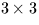Next: SLA_PREC - Precession Matrix (FK5)
Up: SUBPROGRAM SPECIFICATIONS
Previous: SLA_POLMO - Polar Motion

## SLA_PREBN - Precession Matrix (FK4)

ACTION:
Generate the matrix of precession between two epochs, using the old, pre IAU 1976, Bessel-Newcomb model, in Andoyer's formulation.

CALL:
CALL sla_PREBN (BEP0, BEP1, RMATP)

GIVEN:

 D BEP0 beginning Besselian epoch BEP1 D ending Besselian epoch

RETURNED:

 D(3,3) RMATP precession matrix

NOTE:
The matrix is in the sense:
v1 = Mv0
where v1 is the star vector relative to the mean equator and equinox of epoch BEP1, M is thematrix RMATP and v0 is the star vector relative to the mean equator and equinox of epoch BEP0.

REFERENCE:
Smith et al., 1989. Astr.J. 97, 269.Next: SLA_PREC - Precession Matrix (FK5)
Up: SUBPROGRAM SPECIFICATIONS
Previous: SLA_POLMO - Polar Motion

SLALIB --- Positional Astronomy Library